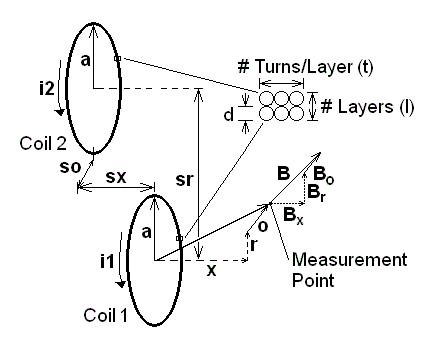Calculator for Off-Axis Magnetic Fields due to One or Two Coils Copyright © 2014 Eric Dennison and Samuel M. Goldwasser, all Rights ReservedThis JavaScript calculator started out using Eric Dennison's "Calculator for Off-Axis Fields due to a Current Loop" found on the "Magnet Formulas" Web site (link below). It was modified by me (Sam) to use units of inches instead of meters (OK, so I'm old-fashioned or something. but inches are more convenient for what I'm doing) and two coils wound using multiple layers with multiple turns and wire of finite diameter. This includes the special cases of solenoid (l=1) and pancake coils (t=1). The separation also allows for the special cases of the Helmholtz (sr=0) and figure-eight (sx=0). The math may not be precisely exact but should be close unless I screwed up a sign or two. ;-) Enter the mean radius of the coils, current in coil 1 (i1) and the relative current in coil 2 (i2 = the value multiplied by i1); total number of layers (l), number of turns/layer (t), and wire spacing (d); axial separation (sx), radial separateion (sr), offset separation (so); and the axial (x), radial (r), and offset (o) position of the measurement point. Then click "Calculate Magnetic Field Components" to determine the contributions from each coil individually and the net field from both. The coordinate system is Cartesian with x,r,o for the three dimensions. This is for legacy reasons. I may change it someday. :) For a single coil - which would be a common use of this calculator - x (axial) and r (radial) makes the most sense. For 2 coils, r is retained but would be equivalent to the y (vertical) coordinate in the diagram. Offset then stands in for z.
 Currents (amperes): Coil 1 (i1) Coil 2 (i2 relative to i1: 1=i1, 0=0, -1=-i1)

 Coils: Radius (a, inches) Wire Spacing (d, inches) # Layers (l) Turns/Layer (t)

 Separation of Centers of Coils (inches): Axial (sx) Radial (sr) Offset (so)

 Position at Measurement Point (inches): Axial (x) Radial (r) Offset (o)

 Field due to Coil 1 (Tesla): Axial (B1x) Radial (B1r) Offset (B1o)

 Field due to Coil 2 (Tesla): Axial (B2x) Radial (B2r) Offset (B2o)

 Field due to Both Coils (Tesla): Axial (Bx) Radial (Br) Offset (Bo)

 More can be found at Eric Dennison's Magnet Formulas Web Site.